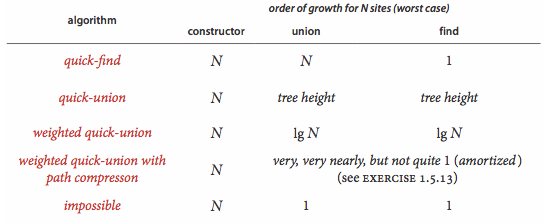# 并查集算法分析

Wafer Li ... 2017-02-04 《Algorithm 第四版》笔记
• Algorithm
• 读书笔记

## # 1. 概念

• 连接

连接是一种等价关系，它意味着以下特点：

• 自反性：$p$ 连接着 $p$
• 对称性：如果 $p$$q$ 相连，那么 $q$$p$ 相连
• 传递性：如果 $p$$q$ 相连，$q$$r$ 相连，那么 $p$$r$ 相连

## # 2. Union-Find 的实现

public static void main(String[] args) {
UF UF = new UF(N);          //Initialize the data structure

while(!StdIn.isEmpty()) {

if(uf.connected(p,q)) {
//If it is connected, ignore it
continue;
}

uf.uinon(p,q);          //Merge the contacts
StdOut.println(p + " " + q);
}
}

1
2
3
4
5
6
7
8
9
10
11
12
13
14
15
16
17

## # 3. union() 和 find() 的实现

### # 3.1 Quick-Find 算法

public int find (int p) {
return id[p];
}

public void union(int p, int q) {
// Merge p and q into the same component
int pID = find(p);
int qID = find(q);

// If the p and q are at the same component,
// do nothing and return.
if(pID = qID) {
retrun;
}

// Rename the p component to the q component
for(int i = 0; i< id.lenth; i++) {
if(id[i] == pID) {
id[i] = qID;
}
}
count --;   // Decrease the component counter
}

1
2
3
4
5
6
7
8
9
10
11
12
13
14
15
16
17
18
19
20
21
22
23

connected() 方法需要 2 次 find()；而对于 union() 方法则需要调用两次 find() ，检查 $N$ 个数组元素，改变其中 $1 \sim N - 1$ 个数组元素的值。

### # 3.2 Quick-Union 算法

#### # 3.2.1 基本概念

1. 使用树作为基本的数据结构

2. 每一个节点的 id[] 元素就是另一个节点的名字，我们认为它们之间建立了一个连接

例如， $p$$q$ 是两个节点，如果 $p$$q$ 连接，那么 id[p] == q

3. 如果 id[p] == p，那么我们称 $p$ 是一个根节点

#### # 3.2.2 实现

private int find(int p) {
// Find the root of the contact's component
while (p != id[p]) {
p = id[p];
}
return p;
}

public void union(int p, int q) {
// Merge the root contact of the p's component and the q's component
int pRoot = find(p);
int qRoot = find(q);

// If the two components' root are the same, return.
if (pRoot == qRoot) {
return;
}

// Set the p's tree links with q's tree.
// Now, the qRoot is the father contact of the qRoot.
id[pRoot] = qRoot;

count --;
}

1
2
3
4
5
6
7
8
9
10
11
12
13
14
15
16
17
18
19
20
21
22
23
24

## # 4. 加权的并查集算法

### # 4.1 实现

public class WeightedQuickUnionUF {

/**
* We need a new array to count the tree's size
* The index is the root contact
* The value is the corresponding size of the tree

* Baically, we use the root contact to stand for the tree
*/
private int[] sz;
....
public WeightedQuickUnionUF (int N) {
...
sz = new int[N];
for (int i = 0; i < N; i++) {
// Initialize the sz[] as 1.
// No one was linked at the first.
sz[i] = 1;
}
}
...
public int find(int p) {
// Find the root contact
while (p != id[p]) {
p = id[p];
}
retrun p;
}

public void union(int p, int q) {
...
if (sz[i] < sz[j]) {    // Link the smaller tree's root contact to the bigger one
id[i] = j;
sz[j] += sz[i];     // Adding the weight(or size) of the component
}
else {
id[j] = i;
sz[i] += sz[j];
}
}
}

1
2
3
4
5
6
7
8
9
10
11
12
13
14
15
16
17
18
19
20
21
22
23
24
25
26
27
28
29
30
31
32
33
34
35
36
37
38
39
40
41

## # 5. 路径压缩的并查集算法

### # 5.1 实现 1 ——两个循环

public int find(int p) {
int pParent = p;
int pRoot = p;

// Find the p's root
while(pRoot != id[pRoot]) {
pRoot = id[pRoot];
}

while (id[p] != p) {
pParent = id[p];
id[p] = pRoot;
p = pParent;
}

return pRoot;
}

1
2
3
4
5
6
7
8
9
10
11
12
13
14
15
16
17

### # 5.2 实现 2 ——将点指向其爷爷节点

public int find(int p) {
while(p != id[p]) {
id[p] = id[id[p]];
p = id[p];
}
}

1
2
3
4
5
6

## # 6. 比较1. 在算法中 $logN$$log_2{N}$ 等价 ↩︎